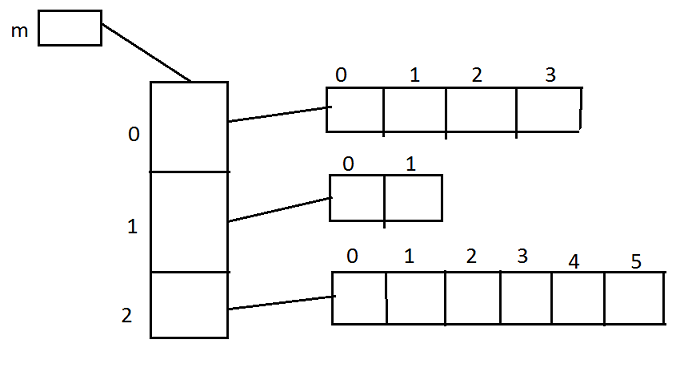# Java Programing laungage

Uneven/Jagged array in java
 Previous Home Next

Jagged arrays in java are arrays containing arrays of different length. Jagged arrays are also multidimensional arrays. Jagged arrays in java sometimes are also called as ragged arrays/uneven array. Ragged array also known as jagged arrays are multi dimensional arrays (though we mostly deal with 2d jagged arrays) in which at least one array doesnt have the same length as the others in the same dimension. Uneven array is an array with more than one dimension each dimension has different size .

### Example :

```int m[][];

m=new int[];

m=new int;
m=new int;
m=new int;``````//program

public class Main
{
public static void main(String args[])
{
int a[][] = new int[];
a = new int;
a = new int;
a = new int;
a = new int;

for (int i = 0; i < 4; i++)
{
for (int j = 0; j < i + 1; j++)
{
a[i][j] = i + j;
}
}
for (int i = 0; i < 4; i++)
{
for (int j = 0; j < i + 1; j++)
System.out.print(twoD[i][j] + " ");
System.out.println();
}
}
}```

### Output :

```0
1 2
2 3 4
3 4 5 6 ```
 Previous Home Next